SSC CGL Previous Year Questions: Algebra- 6

# SSC CGL Previous Year Questions: Algebra- 6 - SSC CGL

Test Description

## 26 Questions MCQ Test SSC CGL (Tier - 1) - Previous Year Papers (Topic Wise) - SSC CGL Previous Year Questions: Algebra- 6

SSC CGL Previous Year Questions: Algebra- 6 for SSC CGL 2023 is part of SSC CGL (Tier - 1) - Previous Year Papers (Topic Wise) preparation. The SSC CGL Previous Year Questions: Algebra- 6 questions and answers have been prepared according to the SSC CGL exam syllabus.The SSC CGL Previous Year Questions: Algebra- 6 MCQs are made for SSC CGL 2023 Exam. Find important definitions, questions, notes, meanings, examples, exercises, MCQs and online tests for SSC CGL Previous Year Questions: Algebra- 6 below.
Solutions of SSC CGL Previous Year Questions: Algebra- 6 questions in English are available as part of our SSC CGL (Tier - 1) - Previous Year Papers (Topic Wise) for SSC CGL & SSC CGL Previous Year Questions: Algebra- 6 solutions in Hindi for SSC CGL (Tier - 1) - Previous Year Papers (Topic Wise) course. Download more important topics, notes, lectures and mock test series for SSC CGL Exam by signing up for free. Attempt SSC CGL Previous Year Questions: Algebra- 6 | 26 questions in 26 minutes | Mock test for SSC CGL preparation | Free important questions MCQ to study SSC CGL (Tier - 1) - Previous Year Papers (Topic Wise) for SSC CGL Exam | Download free PDF with solutions
 1 Crore+ students have signed up on EduRev. Have you?
SSC CGL Previous Year Questions: Algebra- 6 - Question 1

### If x = 2+√3, then the value of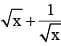is:        (SSC CGL 2nd Sit. 2012)

Detailed Solution for SSC CGL Previous Year Questions: Algebra- 6 - Question 1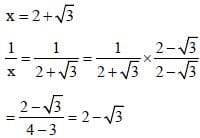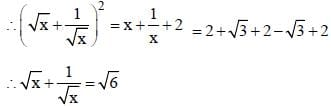SSC CGL Previous Year Questions: Algebra- 6 - Question 2

### If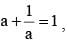then the value of a3 is:       (SSC CGL  2nd Sit. 2012)

Detailed Solution for SSC CGL Previous Year Questions: Algebra- 6 - Question 2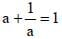a2 + 1 = a
a2 – a + 1 = 0
Multiplying both side by (a + 1)
(a + 1) (a2 – a + 1) = 0
a3 + 1
a3 = –1

SSC CGL Previous Year Questions: Algebra- 6 - Question 3

### If ax + by = 6, bx – ay = 2 and x2 + y2 = 4, then the value of (a2 + b2) would be:        (SSC CGL 2nd Sit. 2012)

Detailed Solution for SSC CGL Previous Year Questions: Algebra- 6 - Question 3

ax + by = 6 ...(i)
bx – ay = 2 ...(ii)
a2x2 + b2y2 + 2abxy + b2x2 + a2y2 – 2abxy
= 36 + 4
⇒ x2 (a2 + b2) + y2 (a2 + b2) = 40
⇒ (a2 + b2) (x2 + y2) = 40
⇒ (a2 + b2) × 4 = 40
⇒ a2 + b2 = 10

SSC CGL Previous Year Questions: Algebra- 6 - Question 4

If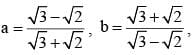then the value of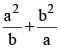is:       (SSC CGL 2nd Sit. 2012)

Detailed Solution for SSC CGL Previous Year Questions: Algebra- 6 - Question 4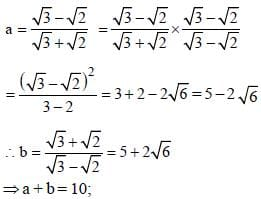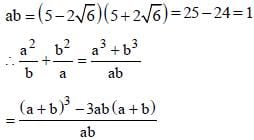= 103 – 3 × 10 = 1000 – 30 = 970

SSC CGL Previous Year Questions: Algebra- 6 - Question 5

If (5x2 – 3y2) : xy = 11 : 2, then the postive value of x/y is:       (SSC CGL 2nd Sit. 2012)

Detailed Solution for SSC CGL Previous Year Questions: Algebra- 6 - Question 5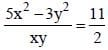10x2 – 6y2 = 11xy
10x2 – 11xy – 6y2 = 0
10x2 – 15xy + 4xy – 6y2 = 0
5x(2x – 3y) + 2y(2x – 3y) = 0
(5x + 2y) (2x – 3y)
5x ≠ 2y, 2x = 3y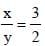SSC CGL Previous Year Questions: Algebra- 6 - Question 6

If (a – b) = 3, (b – c) = 5 and (c – a) = 1, then the value of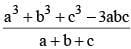is      (SSC CGL  1st Sit. 2012)

Detailed Solution for SSC CGL Previous Year Questions: Algebra- 6 - Question 6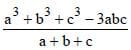a3 + b3 + c3 – 3abc=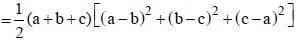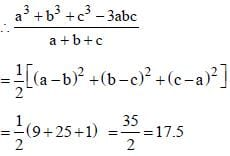SSC CGL Previous Year Questions: Algebra- 6 - Question 7

In the xy–coordinate system, if (a, b) and (a + 3, b + k) are two points on the line defined by the equation x = 3y – 7, then k = ?        (SSC CGL  1st Sit. 2012)

Detailed Solution for SSC CGL Previous Year Questions: Algebra- 6 - Question 7

Points (a, b) and [(a + 3), (b + k)] will satisfy the equation
x – 3y + 7 = 0.
∴ a – 3b + 7 = 0 ......(i)
and a + 3 – 3 (b + k) + 7 = 0
⇒ a + 3 – 3b – 3 k + 7 = 0
⇒ a – 3b + 7 + 3 – 3 k = 0
⇒ 3 – 3k = 0 ⇒ 3k = 3
⇒ k = 3/3 = 1 [∵ a - 3b + 7 = 0]

SSC CGL Previous Year Questions: Algebra- 6 - Question 8

If a + b + 1 = 0, then the value of (a3 + b3 + 1 – 3ab) is        (SSC CGL 1st Sit. 2012)

Detailed Solution for SSC CGL Previous Year Questions: Algebra- 6 - Question 8

If a + b + c = 0
then a3 + b3 + c3 – 3abc = 0
Now,
a + b + 1 = 0
∴ a3 + b3 + 1 – 3ab = 0

SSC CGL Previous Year Questions: Algebra- 6 - Question 9

If x + (1/x) = 3, the value of x5 + (1/)x5)) is      (SSC CGL  1st Sit. 2012)

Detailed Solution for SSC CGL Previous Year Questions: Algebra- 6 - Question 9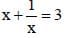On squaring,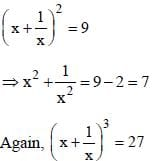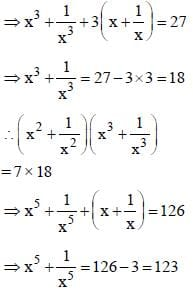SSC CGL Previous Year Questions: Algebra- 6 - Question 10

If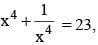then the value of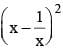will be       (SSC CGL  1st Sit. 2012)

Detailed Solution for SSC CGL Previous Year Questions: Algebra- 6 - Question 10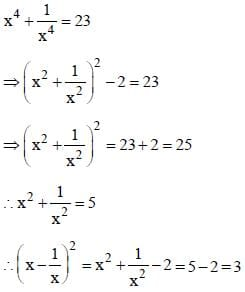SSC CGL Previous Year Questions: Algebra- 6 - Question 11

In xy–plane, P and Q are two points having co–ordinates (2, 0) and (5, 4) respectively. Then the numerical value of the area of the circle with radius PQ is        (SSC CGL  1st Sit. 2012)

Detailed Solution for SSC CGL Previous Year Questions: Algebra- 6 - Question 11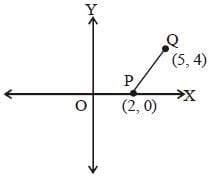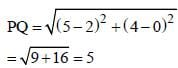∴ Area of circle = πr2
= 25 π sq. units

SSC CGL Previous Year Questions: Algebra- 6 - Question 12

If x = (0.08)2, y =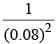and z = (1 – 0.08)2 – 1, then out of the following the true relation is      (SSC CGL 1st Sit. 2012)

Detailed Solution for SSC CGL Previous Year Questions: Algebra- 6 - Question 12

x = (0.08)2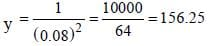Z = (1 – 0.08)2 – 1
= 1 + (0.08)2 – 2 × 0.08 – 1 = (0.08)2 – 2 × 0.08
Clearly, z < x < y

SSC CGL Previous Year Questions: Algebra- 6 - Question 13

The sum of two numbers is 24 and their product is 143. The sum of their squares is      (SSC CGL 2nd Sit. 2011)

Detailed Solution for SSC CGL Previous Year Questions: Algebra- 6 - Question 13

Let the two numbers be x and y.
∴ x + y = 24
and, xy = 143
∴ x2 + y2 = (x + y)2 – 2xy
= (24)2 – 2 × 143 = 576 – 286 = 290

SSC CGL Previous Year Questions: Algebra- 6 - Question 14

If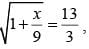then the value of x is      (SSC CGL  2nd Sit. 2011)

Detailed Solution for SSC CGL Previous Year Questions: Algebra- 6 - Question 14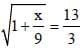Squaring on both sides,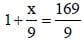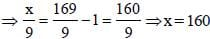SSC CGL Previous Year Questions: Algebra- 6 - Question 15

If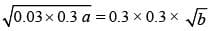value of a/b is     (SSC CGL  2nd Sit. 2011)

Detailed Solution for SSC CGL Previous Year Questions: Algebra- 6 - Question 15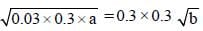On squaring,
0.03 × 0.3 × a = 0.09 × 0.09 × b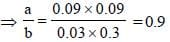SSC CGL Previous Year Questions: Algebra- 6 - Question 16

If 1.5x = 0.04y, then the value of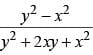is        (SSC CGL  2nd Sit. 2011)

Detailed Solution for SSC CGL Previous Year Questions: Algebra- 6 - Question 16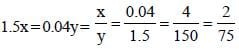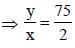Now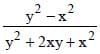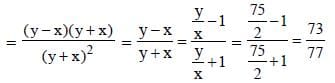SSC CGL Previous Year Questions: Algebra- 6 - Question 17

In an examination a student scores 4 marks for every correct answer and loses 1 mark for every wrong answer. If he attempts all 75 questions and secures 125 marks, the number of questions he attempts correctly is        (SSC CGL 1st Sit. 2011)

Detailed Solution for SSC CGL Previous Year Questions: Algebra- 6 - Question 17

Let the number of correct answers be x.
∴ x + 4 – (75 – x) × 1 = 125
⇒ 4x – 75 + x = 125
⇒ 5x = 125 + 75 = 200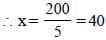SSC CGL Previous Year Questions: Algebra- 6 - Question 18

If p and q represent digits, what is the possible maximum value of q in the statement 5p9 + 327 + 2q8 = 1114?       (SSC CGL  2nd Sit. 2010)

Detailed Solution for SSC CGL Previous Year Questions: Algebra- 6 - Question 18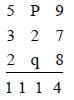If p = 0, then q’s maximum value = 7.

SSC CGL Previous Year Questions: Algebra- 6 - Question 19

It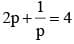the value of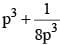is        (SSC CGL 2nd  Sit. 2010)

Detailed Solution for SSC CGL Previous Year Questions: Algebra- 6 - Question 19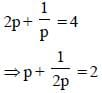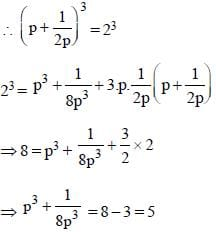SSC CGL Previous Year Questions: Algebra- 6 - Question 20

In an examination, a student scores 4 marks for every correct answer and loses 1 mark for every wrong answer. A student attempted all the 200 questions and scored, in all 200 marks. The number of questions, he answered correctly was         (SSC CGL 2nd Sit. 2010)

Detailed Solution for SSC CGL Previous Year Questions: Algebra- 6 - Question 20

If the number of correct answers be x, then
x × 4 – 1. (200 – x) = 200
⇒ 4x – 200 + x = 200
⇒ 5x = 400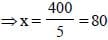SSC CGL Previous Year Questions: Algebra- 6 - Question 21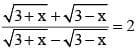then x is equal to      (SSC CGL 1st Sit. 2010)

Detailed Solution for SSC CGL Previous Year Questions: Algebra- 6 - Question 21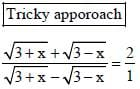By componendo and dividendo,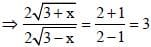Squaring both sides,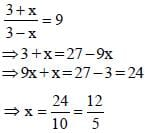SSC CGL Previous Year Questions: Algebra- 6 - Question 22

Two natural numbers are in the ratio 3 : 5 and their product is 2160. The smaller of the numbers is        (SSC CGL 1st Sit. 2010)

Detailed Solution for SSC CGL Previous Year Questions: Algebra- 6 - Question 22

Let the numbers be 3x and 5x.
∴ 3x × 5x = 2160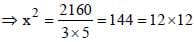⇒ x = 12
∴ Smaller number = 3x = 3 × 12 = 36

SSC CGL Previous Year Questions: Algebra- 6 - Question 23

If a and b be positive integers such that a2 – b2 = 19, then the value of a is      (SSC CGL 2010)

Detailed Solution for SSC CGL Previous Year Questions: Algebra- 6 - Question 23

a2 – b2 = 19
⇒ 102 – 92 = 19
⇒ a = 10

SSC CGL Previous Year Questions: Algebra- 6 - Question 24

If a = 11 and b = 9, then the value of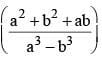is       (SSC CGL 1st Sit. 2010)

Detailed Solution for SSC CGL Previous Year Questions: Algebra- 6 - Question 24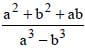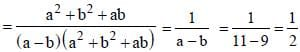SSC CGL Previous Year Questions: Algebra- 6 - Question 25

If a and b are two odd positive integers, by which of the following integers is (a4 – b4) always divisible?      (SSC CGL 1st Sit. 2010)

Detailed Solution for SSC CGL Previous Year Questions: Algebra- 6 - Question 25

a4 – b4 = (a2 + b2) (a + b) (a – b)
∴ Required number = (3 + 1) ( 3 – 1) = 8

SSC CGL Previous Year Questions: Algebra- 6 - Question 26

If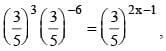then x is equal to       (SSC CGL 1st Sit. 2010)

Detailed Solution for SSC CGL Previous Year Questions: Algebra- 6 - Question 26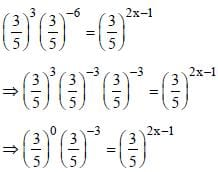⇒ 2x – 1 = –3
⇒ 2x = –3 + 1 = –2
⇒ x = –1

## SSC CGL (Tier - 1) - Previous Year Papers (Topic Wise)

250 tests
Information about SSC CGL Previous Year Questions: Algebra- 6 Page
In this test you can find the Exam questions for SSC CGL Previous Year Questions: Algebra- 6 solved & explained in the simplest way possible. Besides giving Questions and answers for SSC CGL Previous Year Questions: Algebra- 6, EduRev gives you an ample number of Online tests for practice

250 tests